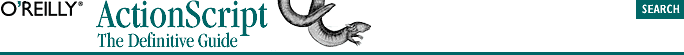home | O'Reilly's CD bookshelfs | FreeBSD | Linux | Cisco | Cisco Exam## 12.4. Methods

`objectName.methodName( )`

For example:

`ball.getArea( );  // Call the getArea( ) method of ball`

As with properties, methods are defined for a class and then invoked on individual object instances. However, before we see how to create methods within a class, we're going to bend the rules of good object-oriented programming and attach a method to an individual object instance of the built-in Object class. Once we understand how a single method works on an isolated object, we'll apply the concept correctly to a class of our own.

A method is essentially just a function stored in an object property. By assigning a function to an object property, we turn that function into a method of the object:

```// Create an object
myObject = new Object( );

// Create a function
function greet ( ) {
trace("hello world");
}

// Now assign greet to the property sayHello
myObject.sayHello = greet;```

Once a function resides in an object property, we may invoke that function as a method, like this:

`myObject.sayHello( );  // Displays: "hello world"`

Truth be known, you can also assign a function to an array element (instead of to an object property) and invoke that function using the call operator, like this:

```var myList = new Array( );
myList = greet;
myList( );          // Displays: "hello world"```
`myObject.msg = "Nice day, isn't it?";`

Now we adjust greet( ) so that it displays the value of msg:

```function greet ( ) {
trace(this.msg);
}

// Now invoke sayHello again
myObject.sayHello( );  // Displays: "Nice day, isn't it?"```

Notice the keyword this at the end of line 2? That's our connection to myObject. When executed as a method of myObject, sayHello( ) is effectively passed an invisible argument called this containing a reference to myObject. In the body of the function, we use this to access myObject's msg property as this.msg. When we invoke myObject.sayHello( ), the expression this.msg resolves to myObject.msg, which becomes "Nice day, isn't it?".

A method can both retrieve and set the value of its host object's properties. Example 12-1 demonstrates a method that retrieves the value of two properties of an object, performs a calculation based on those values, and then sets the value of a third, new property. (Once again, because our current focus is methods, the object we're using is only an object instance. In the next section we'll learn how to add object methods properly with a class.)

#### Example 12-1. Implementing a Method that Sets a Property

```// Make a new object and store it in rectangle
var rectangle = new Object( );
rectangle.width = 10;
rectangle.height = 5;

// Define a function to calculate a rectangle's area, and
// set a corresponding property. Note the use of the this keyword.
function rectArea( ) {
this.area = this.width * this.height;
}

// Assign the function as a method of rectangle
rectangle.setArea = rectArea;

// Now invoke rectangle's setArea( ) method
rectangle.setArea( );    // Sets rectangle.area to 50

// Finally, examine the new property generated by setArea( )
trace(rectangle.area);  // Displays: 50```

#### Example 12-2. Returning a Value from a Method

```// Create and set up our rectangle. Note that it's legal to assign
// the area method before the rectArea function appears in our code.
var rectangle = new Object( );
rectangle.width = 10;
rectangle.height = 5;
rectangle.area = rectArea;

// This time, just return the area, don't assign it to a property
function rectArea( ) {
return this.width * this.height;
}

// Now it's even easier to use
trace("The area of rectangle is: " + rectangle.area( ));

// Displays: "The area of rectangle is: 50"```

The this keyword can be a tricky concept because it involves some sleight of hand by the interpreter. To illustrate how the preceding code works, consider Example 12-3, which exposes the implied relationship of this to its object and method. (Example 12-3 is fictitious because this is a reserved keyword and would cause an error if used as shown.)

#### Example 12-3. A Fictitious Example Using this Explicitly

```// Create and set up our rectangle object
var rectangle = new Object( );
rectangle.width = 10;
rectangle.height = 5;
rectangle.area = rectArea;

// Specifically require this as an argument of our rectArea( ) function
function rectArea(this) {
return this.width * this.height;
}

// Call the method with an explicit reference to the rectangle object
trace("The area of rectangle is: " + rectangle.area(rectangle));```

Methods may also be assigned to object properties as function literals. By using a function literal to create a method, we avoid the work of first creating our function and then assigning it to a property. Example 12-4 reworks our rectangle object from Example 12-2 showing the use of a function literal.

#### Example 12-4. Implementing Methods with Function Literals

```var rectangle = new Object( );
rectangle.width = 10;
rectangle.height = 5;

// Here's the function literal assignment
rectangle.area = function ( ) {
return this.width * this.height;
};  // Using a semicolon is good form at the end of the literal

// We can still invoke the function as before
trace("The area of rectangle is: " + rectangle.area( ));```

Methods give us enormous control over objects. When used with built-in objects, methods are one of our primary tools for controlling the Flash movie environment.

In this section we've seen both how to store a function in a property (thereby making it a method) and also how to invoke a function through a property. These two endeavors have very similar syntax, but very different results. It's crucial to understand the difference.

#### TIP

`myObj.myMethod = someFunction;`

When invoking a method, include the parentheses:

`myObj.myMethod( );`